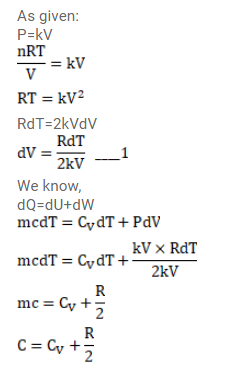# An ideal gas is taken through a process inQuestion:

An ideal gas is taken through a process in which the pressure and the volume are changed according to

the equation $p=k V$. Show that the molar heat capacity of the gas for the process is given by $C=C_{v}+\frac{R}{2}$.

Solution: